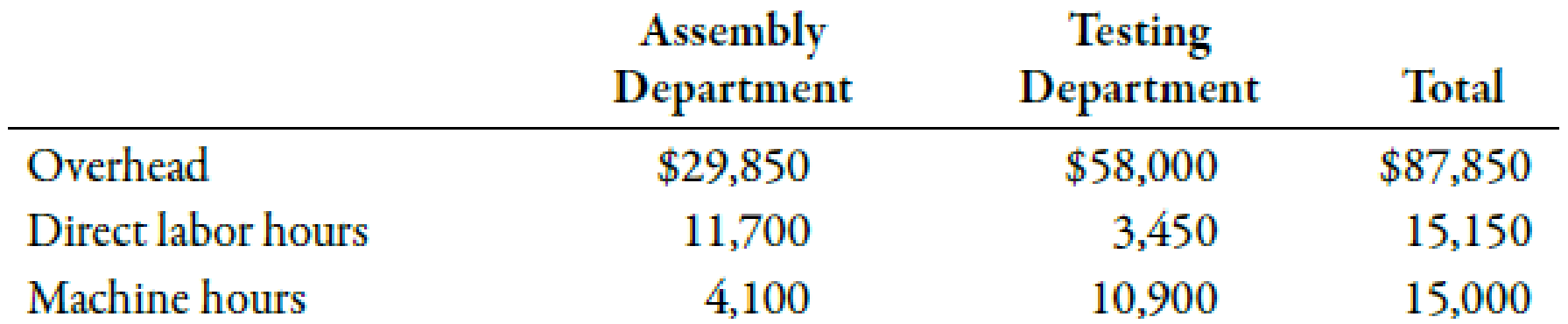Chapter 4, Problem 40E### Managerial Accounting: The Corners...

7th Edition
Maryanne M. Mowen + 2 others
ISBN: 9781337115773

#### Solutions

Chapter
Section### Managerial Accounting: The Corners...

7th Edition
Maryanne M. Mowen + 2 others
ISBN: 9781337115773
Textbook Problem
1 views

# Calculating Departmental Overhead Rates and Applying Overhead to ProductionAt the beginning of the year, Glaser Company estimated the following:Glaser uses departmental overhead rates. In the assembly department, overhead is applied on the basis of direct labor hours. In the testing department, overhead is applied on the basis of machine hours. Actual data for the month of March are as follows:Required: 1. Calculate the predetermined overhead rates for the assembly and testing departments. 2. Calculate the overhead applied to production in each department for the month of March. 3. By how much has each department’s overhead been overapplied? Underapplied?

1.

To determine

Calculate the predetermined overhead rate for the assembly and testing departments.

Explanation

The amount which is calculated at the beginning of the accounting year for a related activity by dividing the total estimated annual overhead by estimated annual activity level is known as the predetermined overhead rate.

Use the following formula to calculate predetermined overhead rate assembly department:

Substitute $338,000 for estimated annual overhead and 130,000 direct labor hours (DLH) for estimated annual activity level in the above formula. Assemblydepartmentoverheadrate=$338,000130,000DLH=2.6

Therefore, assembly department overhead rate is 2

2.

To determine

Calculate the overhead applied to production in each department for the month of March.

3.

To determine

Calculate the amount of overapplied or underapplied overhead for each department.

### Still sussing out bartleby?

Check out a sample textbook solution.

See a sample solution

#### The Solution to Your Study Problems

Bartleby provides explanations to thousands of textbook problems written by our experts, many with advanced degrees!

Get Started